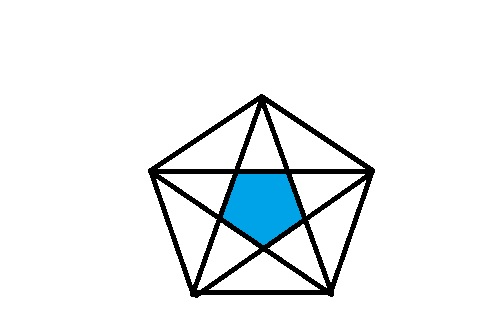Double Trouble

Geometry Level 5The alternate (interior) angles of a regular pentagon are joined to form another regular pentagon. The ratio of the area of the smaller pentagon to the area of the bigger pentagon in simplest form is $( a - \sqrt{b} \times c ) : d,$ where $a ,b ,c , d$ all are pairwise co-prime and $a>0.$ Find $a + b + c + d.$

×